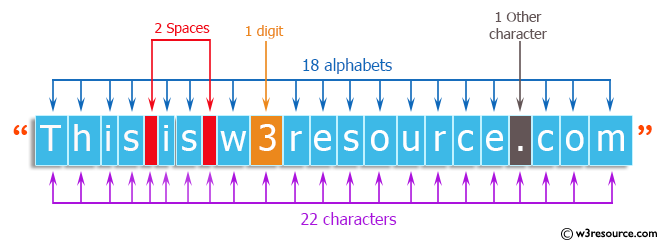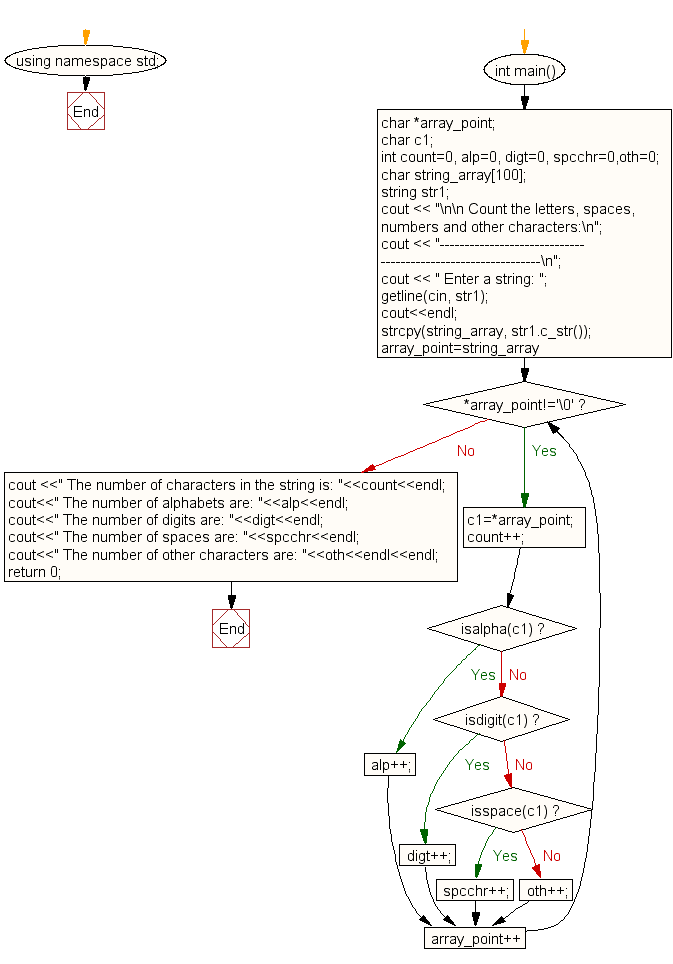﻿ C++ : Count the letters, spaces, numbers of a string# C++ Exercises: Count the letters, spaces, numbers and other characters of an input string.

## C++ For Loop: Exercise-86 with Solution

Write a program in C++ to count the letters, spaces, numbers and other characters of an input string.

Pictorial Presentation:Sample Solution:-

C++ Code :

``````#include<iostream>
#include<string>
#include<cstring>
using namespace std;

int main()
{
char *array_point;
char c1;
int count=0, alp=0, digt=0, spcchr=0,oth=0;
char string_array;
string str1;

cout << "\n\n Count the letters, spaces, numbers and other characters:\n";
cout << "-------------------------------------------------------------\n";
cout << " Enter a string: ";
getline(cin, str1);
cout<<endl;
strcpy(string_array, str1.c_str());
for(array_point=string_array;*array_point!='\0';array_point++)
{
c1=*array_point;
count++;
if (isalpha(c1))
{
alp++;
}
else
if (isdigit(c1))
{
digt++;
}
else
if (isspace(c1))
{
spcchr++;
}
else
{
oth++;;
}
}
cout <<" The number of characters in the string is: "<<count<<endl;
cout<<" The number of alphabets are: "<<alp<<endl;
cout<<" The number of digits are: "<<digt<<endl;
cout<<" The number of spaces are: "<<spcchr<<endl;
cout<<" The number of other characters are: "<<oth<<endl<<endl;
return 0;
}
``````

Sample Output:

``` Count the letters, spaces, numbers and other characters:
-------------------------------------------------------------
Enter a string: This is w3resource.com

The number of characters in the string is: 22
The number of alphabets are: 18
The number of digits are: 1
The number of spaces are: 2
The number of other characters are: 1
```

Flowchart:C++ Code Editor:

Contribute your code and comments through Disqus.

What is the difficulty level of this exercise?

﻿